Home » Wiki » Variable Frequency Drive for Variable Torque Loads

# Variable Frequency Drive for Variable Torque Loads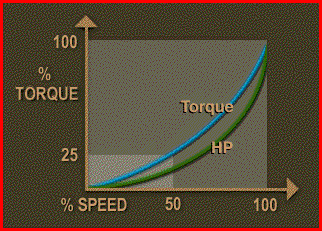Variable torque loads require much lower torque at low speeds than at high speeds. The torque required varies as the square of the speed and the horsepower required varies as the cube of the speed. Variable torque loads include most centrifugal and axial pumps, fans and blowers and many mixers and agitators.

As the speed is decreased, the torque will decrease by the square of the speed decrease and the horsepower required decreases by the cube of the speed decrease.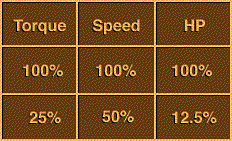As an example, when the speed of a variable torque load is reduced by 50% or one half, the torque required to drive the load is reduced to one-quarter or 25%. The horsepower is reduced to the speed cubed, which is 1/8, or 12.5% of that required to drive the load at full speed. There is an installation example for a variable torque load as following.

A 3 phase, 100 horsepower motor drives a water pump, supplying a plant with certain flow rates. These flow rates vary during the day requiring the motor to operate under varying loads each day. The motor was sized to drive the pump at its maximum flow rate.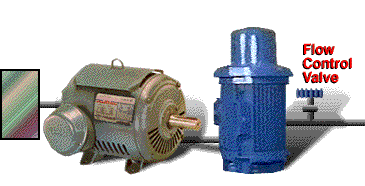The flow rate is controlled by a flow control valve, restricting flow during normal operations. There are no other speed or flow control devices being used.

Is this operation a candidate for a VFD? Several people have suggested addition of a VFD as a way to reduce energy costs and better match needs of the plant to the water being pumped. Let's look at each aspect of this operation in order to make a rational decision about adding a VFD.

Specifications
An existing 3 phase, 100 horsepower motor drives a water pump that has a variable load. The motor operates 20 hours per day. The existing motor nameplate indicates a rated efficiency of 92%.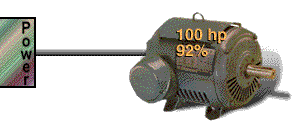Operational Data
Water requirements of the plant vary during the day.
• From 6 a.m. to noon, the plant needs 50% of maximum flow.
• From noon to 12 midnight, the plant needs 75% of maximum flow.
• From 12 midnight to 2 a.m., the plant needs 100% of maximum flow for cleanup.
The motor was sized to drive the pump at the maximum flow rate needed for cleanup operations. A flow control valve is used to reduce the water flow rate to the plant for normal operations.

Energy Costs
The rate for electricity is 4 cents per kilowatt hour for standard energy use and \$5 dollars per peak demand kilowatt each month. The demand is charged on measured usage between 12 noon and 12 midnight.

To evaluate benefits of installing a VFD, a detailed load analysis is required. This means we have to calculate the horsepower requirements for each level of operation.

Because we are dealing with a pumping application, we must obey the affinity laws for fans and pumps. Part of this law states that horsepower varies as the cube of the fan or pump speed variance.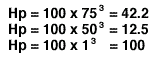Power requirements at 75% flow would require 100 horsepower times 75% cubed,or 42.2 horsepower. At 50% flow the motor would only need to develop 12.5horsepower. At 100% flow, the motor will still develop 100% power, or 100 horsepower.

It is this difference in required horsepower that will make the economic difference if we add the variable frequency drive. The question then becomes, how much will it save?

kW Used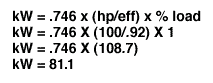The current system's kilowatt usage equals the horsepower (100) divided by the efficiency (92%) times the percent loading (100%) times 0.746 to convert horsepower to kilowatts which equals 81.1 kilowatts.

The yearly energy use equals the kilowatts (81.1 kW) times the operating hours/year, 7,280. The annual energy use is 590,408 kWh.

Annual Energy Costs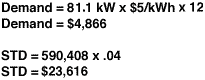Annual energy costs are calculated by taking the demand charge, \$5, times 81.1 kW, times 12 months/year; plus the standard per kilowatt hour rate, \$0.04, times the annual usage of 590,408 kWh. This amounts to \$28,482.

Savings by Adding variable frequency drive
When adding a variable frequency drive to pump, we will assume rated motor efficiency under all loads. As we did previously, we will calculate kilowatt-hours consumed per day at each different horsepower loading and corresponding speed level.

For the 2 hour period where the motor is running at 100%, the annual energy use is 59,041 kWh.

Repeating the calculation for the other two periods (22,058 and 149,386), we see that the annual total usage is 230,485 kWh.

We compute the annual energy cost as before. The annual energy costs of this operation after adding the variable frequency drive equals \$11,271. That gives us an annual energy savings per year of \$17,211.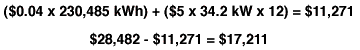Payback
The cost of the variable frequency drive is \$25,000. To find the simple payback, we divide the cost of the variable frequency drive by annual energy savings per year. The simple payback of installing the variable frequency drive is 1.45 years or about 17 months.

Should we install the variable frequency drive? Industrial plant managers will not pay extra money for equipment with payback more than 2 or 3 years. Payback is less than 2 years so the variable frequency drive makes economic sense.

The variable frequency drive has a rated life of 50,000 hours. Dividing 50,000 hours of life by 7,280 hours/year equals a life expectancy of 6.9 years. All in all, this installation makes good economic sense.

Plz Calculate (8 - 6) =
(Your comment will show after approved.)

You may also like:

Category
Featured Articles
Variable frequency drive Rectifier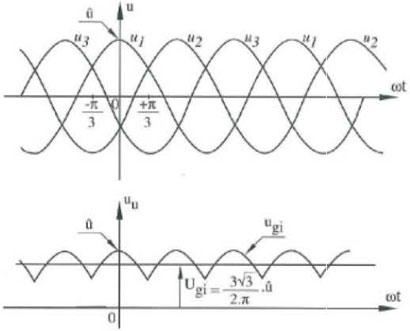To understand variable frequency drive (VFD) better, it's necessary to explain some of the main parts of the variable frequency ...
What is VFD, How it works? - VFD ...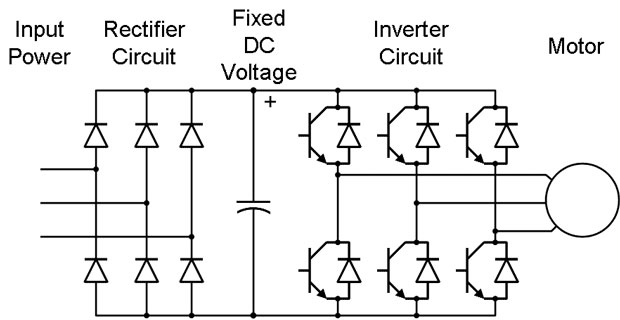VFD is shorted for Variable Frequency Drive (also known as AC Drives and Inverters) -- that's used to make an AC motor working in ...
VFD: Insulated Gate Bipolar Transistor ...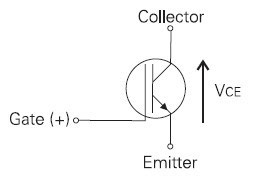IGBT (insulated gate bipolar transistor) provides a high switching speed necessary for PWM VFD operation. IGBTs are capable of ...
VFD controlled Induction motor ...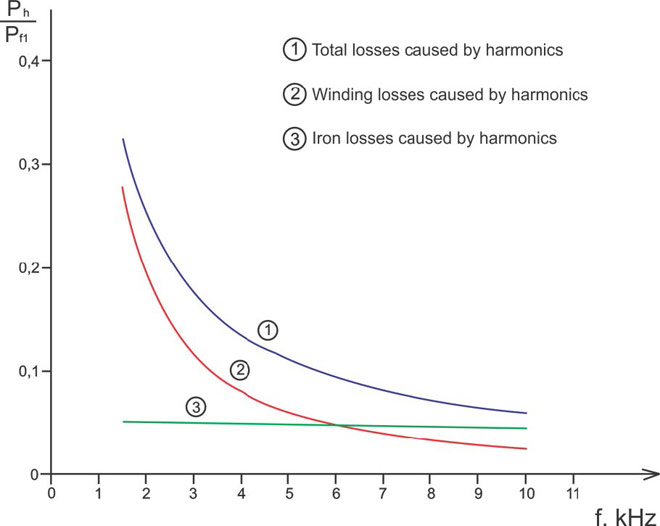This paper presents a procedure to measure the efficiency on an induction motor fed by a VFD by the all operation range to speed ...
Three phase inverters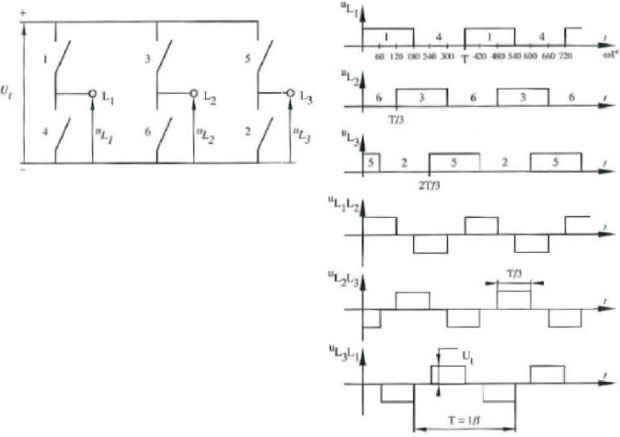In the variable frequency drive rectifier paper, it explains how to go from three phase alternating current voltage to a direct ...VFD manufacturers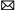buy@vfds.orgtech@vfds.org